Скачать презентацию ECE CS 372 introduction to computer networks Lecture

aaac4c5318a80b551d7ca27c7ab4cae5.ppt

• Количество слайдов: 39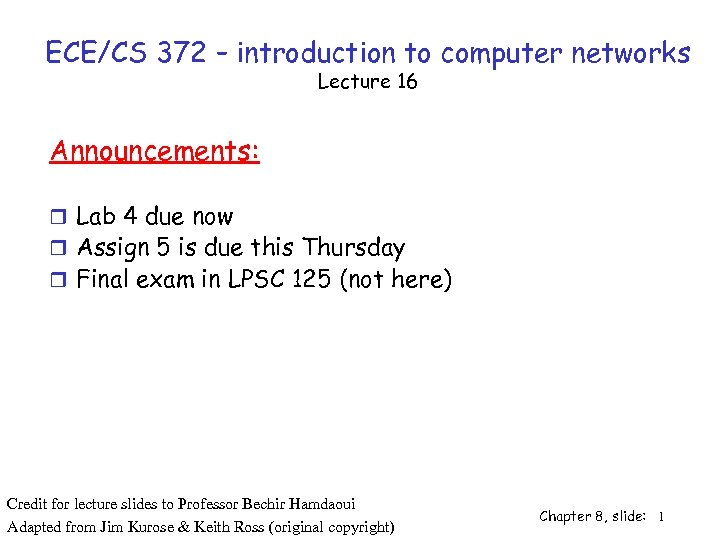ECE/CS 372 – introduction to computer networks Lecture 16 Announcements: r Lab 4 due now r Assign 5 is due this Thursday r Final exam in LPSC 125 (not here) Credit for lecture slides to Professor Bechir Hamdaoui Adapted from Jim Kurose & Keith Ross (original copyright) Chapter 8, slide: 1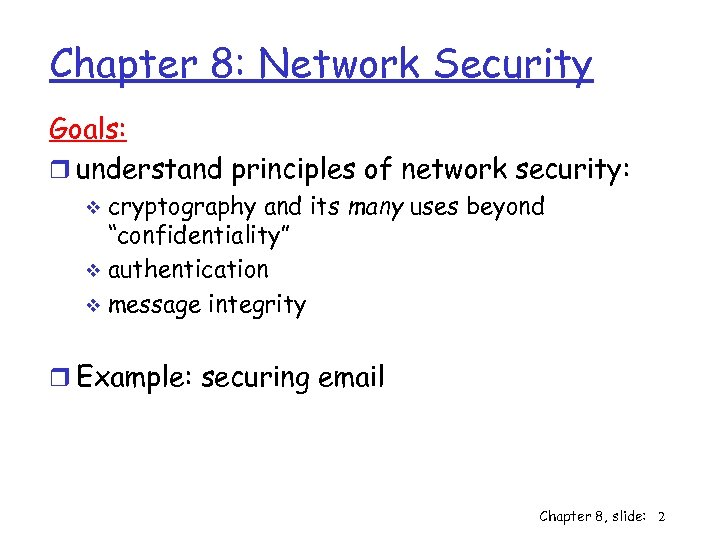Chapter 8: Network Security Goals: r understand principles of network security: cryptography and its many uses beyond “confidentiality” v authentication v message integrity v r Example: securing email Chapter 8, slide: 2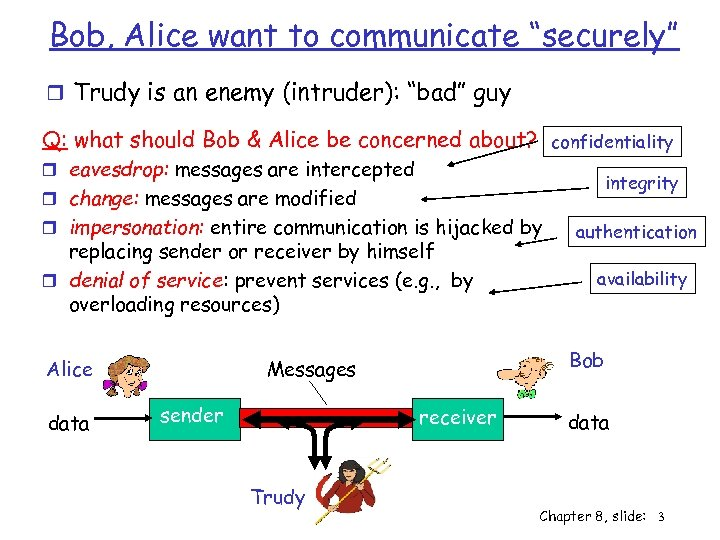Bob, Alice want to communicate “securely” r Trudy is an enemy (intruder): “bad” guy Q: what should Bob & Alice be concerned about? confidentiality r eavesdrop: messages are intercepted integrity r change: messages are modified r impersonation: entire communication is hijacked by replacing sender or receiver by himself r denial of service: prevent services (e. g. , by overloading resources) data sender receiver Trudy availability Bob Messages Alice authentication data Chapter 8, slide: 3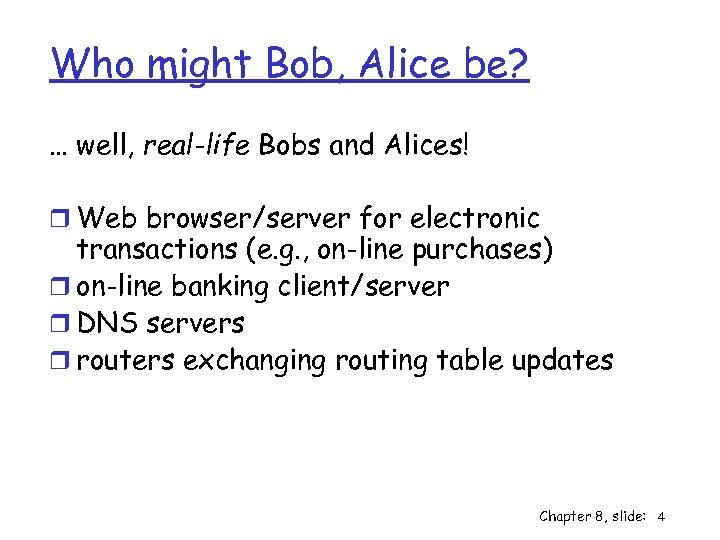Who might Bob, Alice be? … well, real-life Bobs and Alices! r Web browser/server for electronic transactions (e. g. , on-line purchases) r on-line banking client/server r DNS servers r routers exchanging routing table updates Chapter 8, slide: 4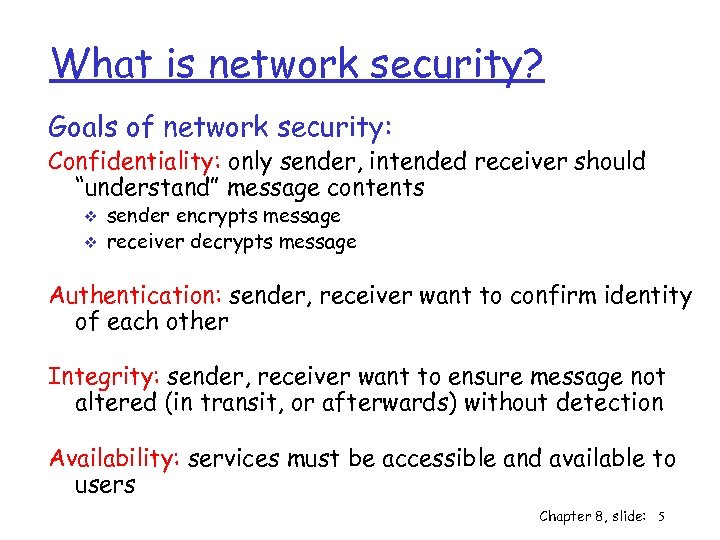What is network security? Goals of network security: Confidentiality: only sender, intended receiver should “understand” message contents v v sender encrypts message receiver decrypts message Authentication: sender, receiver want to confirm identity of each other Integrity: sender, receiver want to ensure message not altered (in transit, or afterwards) without detection Availability: services must be accessible and available to users Chapter 8, slide: 5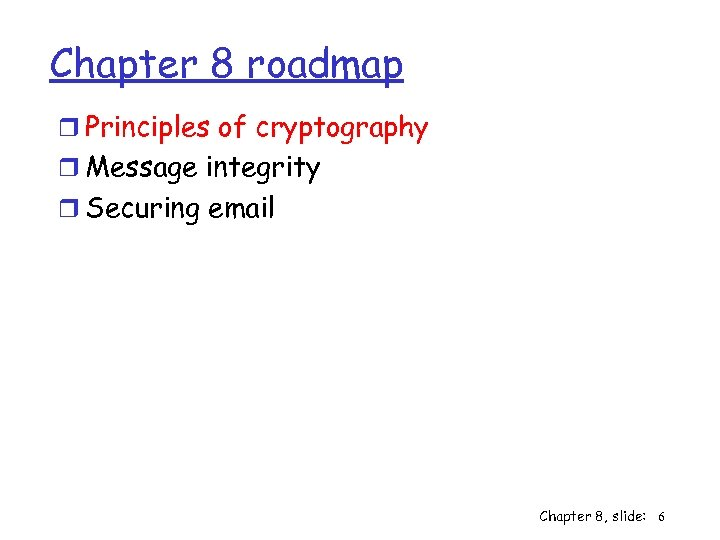Chapter 8 roadmap r Principles of cryptography r Message integrity r Securing email Chapter 8, slide: 6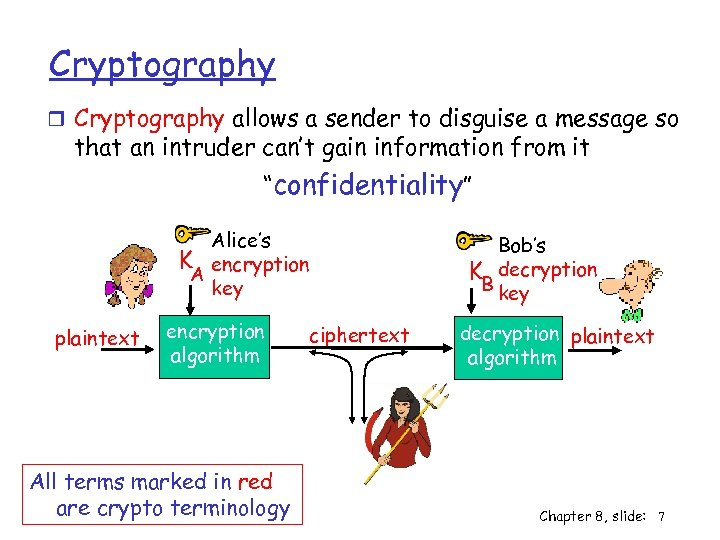Cryptography r Cryptography allows a sender to disguise a message so that an intruder can’t gain information from it “confidentiality” Alice’s K encryption A key plaintext encryption algorithm All terms marked in red are crypto terminology Bob’s K decryption B key ciphertext decryption plaintext algorithm Chapter 8, slide: 7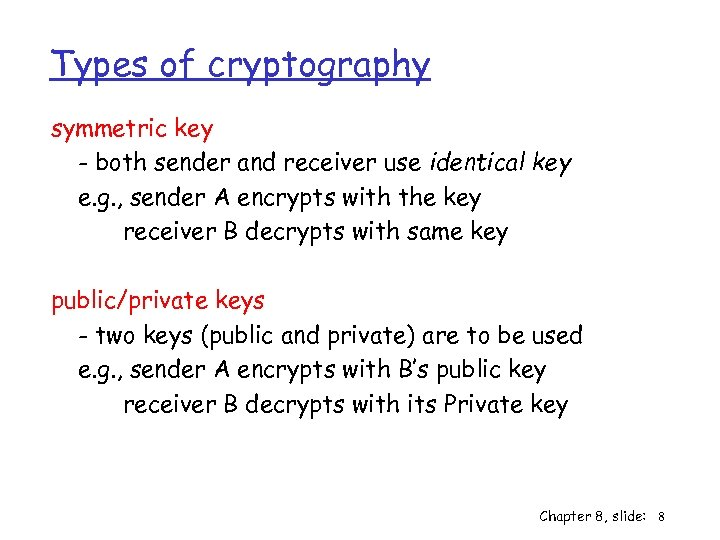Types of cryptography symmetric key - both sender and receiver use identical key e. g. , sender A encrypts with the key receiver B decrypts with same key public/private keys - two keys (public and private) are to be used e. g. , sender A encrypts with B’s public key receiver B decrypts with its Private key Chapter 8, slide: 8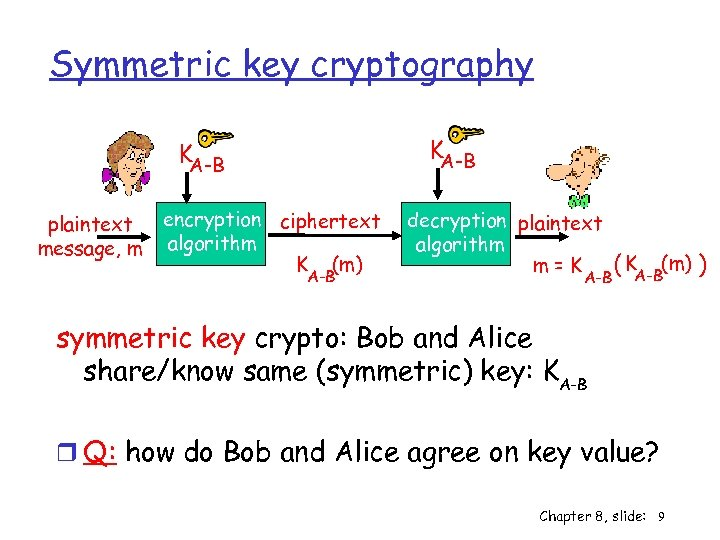Symmetric key cryptography KA-B plaintext message, m encryption ciphertext algorithm K (m) A-B decryption plaintext algorithm m=K A-B ( KA-B(m) ) symmetric key crypto: Bob and Alice share/know same (symmetric) key: KA-B r Q: how do Bob and Alice agree on key value? Chapter 8, slide: 9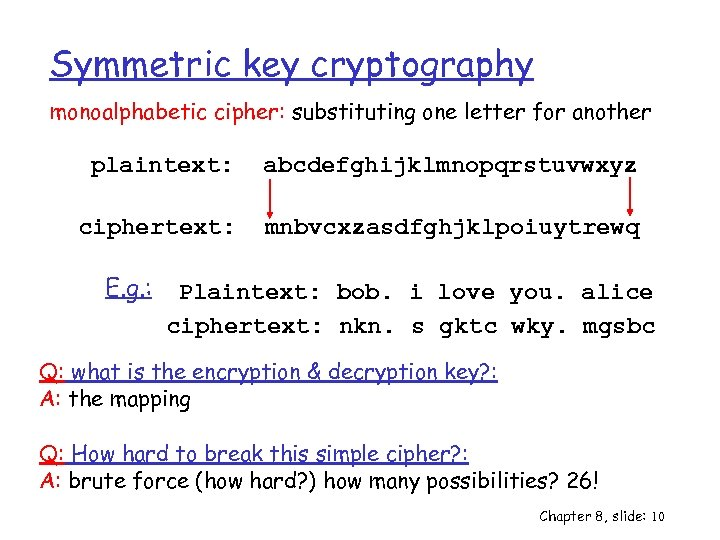Symmetric key cryptography monoalphabetic cipher: substituting one letter for another plaintext: abcdefghijklmnopqrstuvwxyz ciphertext: mnbvcxzasdfghjklpoiuytrewq E. g. : Plaintext: bob. i love you. alice ciphertext: nkn. s gktc wky. mgsbc Q: what is the encryption & decryption key? : A: the mapping Q: How hard to break this simple cipher? : A: brute force (how hard? ) how many possibilities? 26! Chapter 8, slide: 10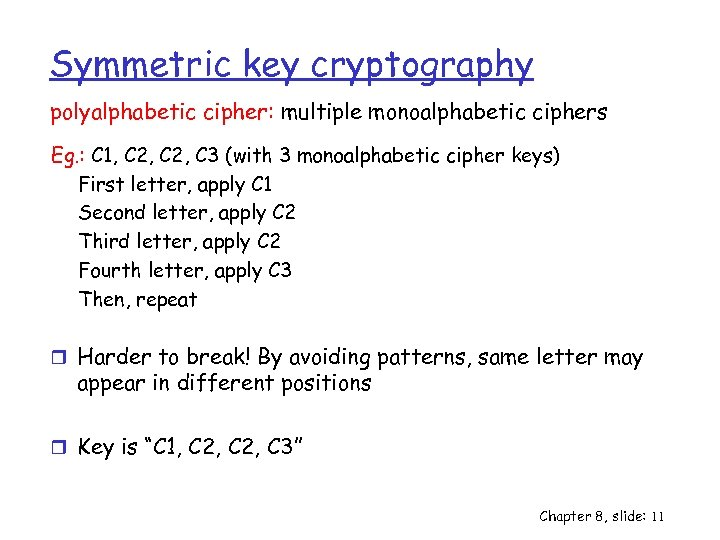Symmetric key cryptography polyalphabetic cipher: multiple monoalphabetic ciphers Eg. : C 1, C 2, C 3 (with 3 monoalphabetic cipher keys) First letter, apply C 1 Second letter, apply C 2 Third letter, apply C 2 Fourth letter, apply C 3 Then, repeat r Harder to break! By avoiding patterns, same letter may appear in different positions r Key is “C 1, C 2, C 3” Chapter 8, slide: 11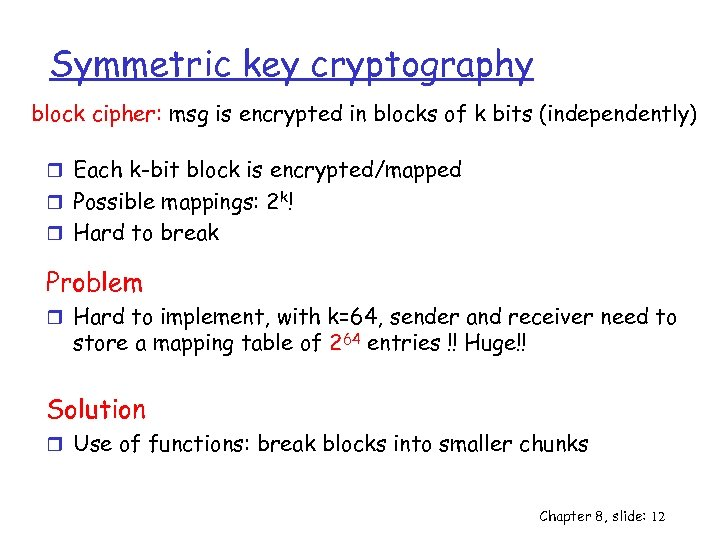Symmetric key cryptography block cipher: msg is encrypted in blocks of k bits (independently) r Each k-bit block is encrypted/mapped r Possible mappings: 2 k! r Hard to break Problem r Hard to implement, with k=64, sender and receiver need to store a mapping table of 264 entries !! Huge!! Solution r Use of functions: break blocks into smaller chunks Chapter 8, slide: 12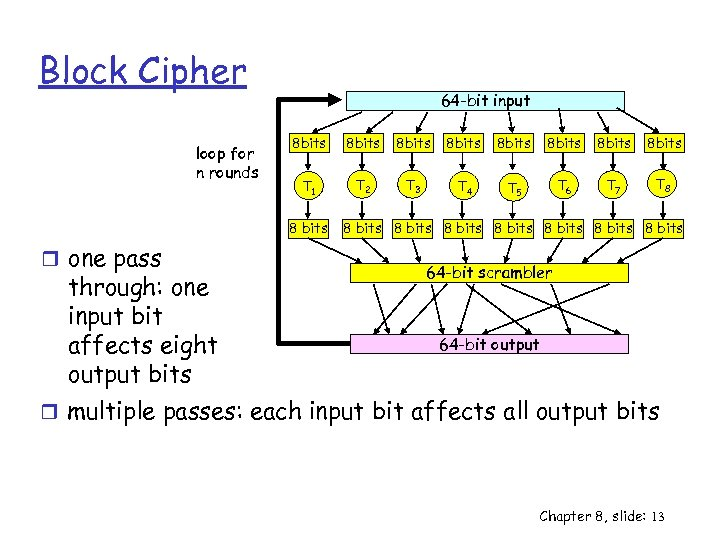Block Cipher loop for n rounds 64 -bit input 8 bits 8 bits T 1 T 2 T 3 T 4 T 5 T 6 T 7 T 8 8 bits r one pass through: one input bit affects eight output bits 8 bits 8 bits 64 -bit scrambler 64 -bit output r multiple passes: each input bit affects all output bits Chapter 8, slide: 13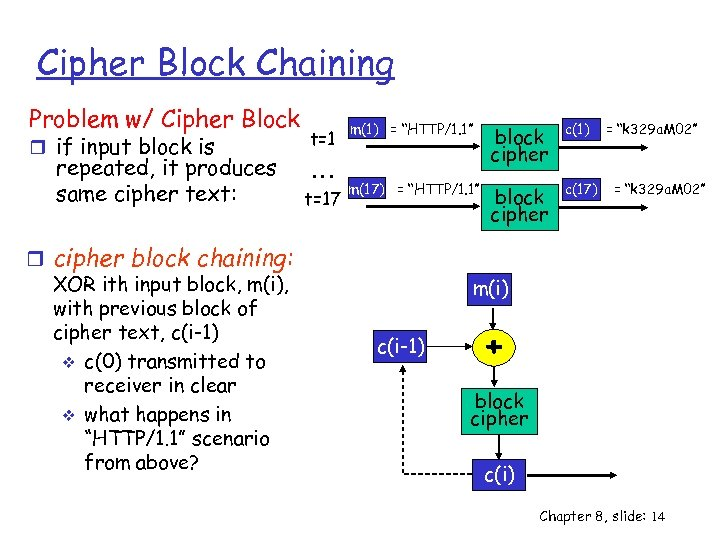Cipher Block Chaining Problem w/ Cipher Block r if input block is repeated, it produces same cipher text: r cipher block chaining: XOR ith input block, m(i), with previous block of cipher text, c(i-1) v c(0) transmitted to receiver in clear v what happens in “HTTP/1. 1” scenario from above? t=1 … t=17 m(1) = “HTTP/1. 1” block cipher c(1) m(17) = “HTTP/1. 1” block cipher c(17) = “k 329 a. M 02” m(i) c(i-1) + block cipher c(i) Chapter 8, slide: 14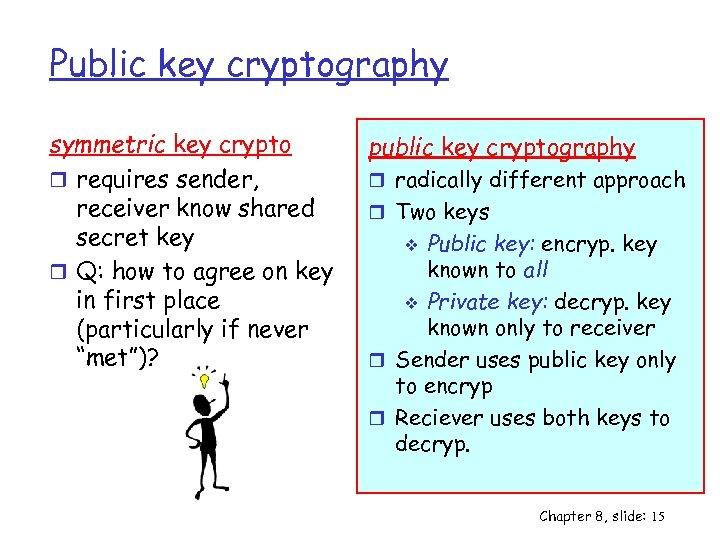Public key cryptography symmetric key crypto r requires sender, receiver know shared secret key r Q: how to agree on key in first place (particularly if never “met”)? public key cryptography r radically different approach r Two keys Public key: encryp. key known to all v Private key: decryp. key known only to receiver r Sender uses public key only to encryp r Reciever uses both keys to decryp. v Chapter 8, slide: 15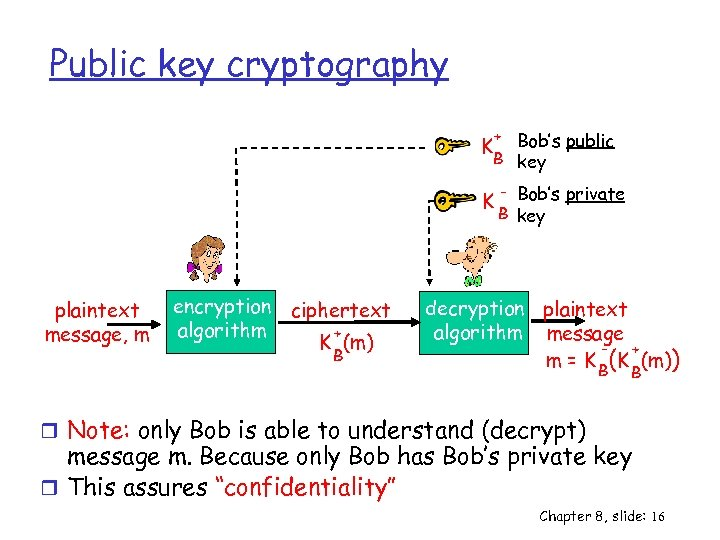Public key cryptography + Bob’s public B key K K plaintext message, m encryption ciphertext algorithm + K (m) B - Bob’s private B key decryption plaintext algorithm message + m = K B(K (m)) B r Note: only Bob is able to understand (decrypt) message m. Because only Bob has Bob’s private key r This assures “confidentiality” Chapter 8, slide: 16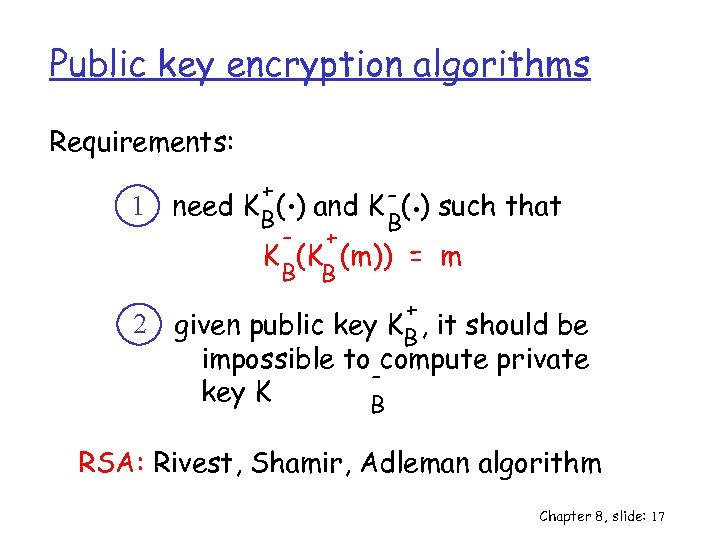Public key encryption algorithms Requirements: 1 2 . . + need K B( ) and K - ( ) such that B - + K (K (m)) = m B B + given public key KB , it should be impossible to compute private key K B RSA: Rivest, Shamir, Adleman algorithm Chapter 8, slide: 17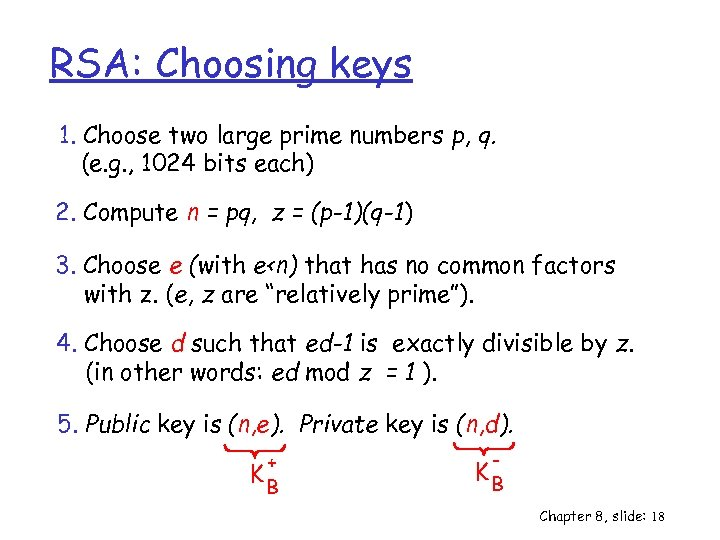RSA: Choosing keys 1. Choose two large prime numbers p, q. (e. g. , 1024 bits each) 2. Compute n = pq, z = (p-1)(q-1) 3. Choose e (with e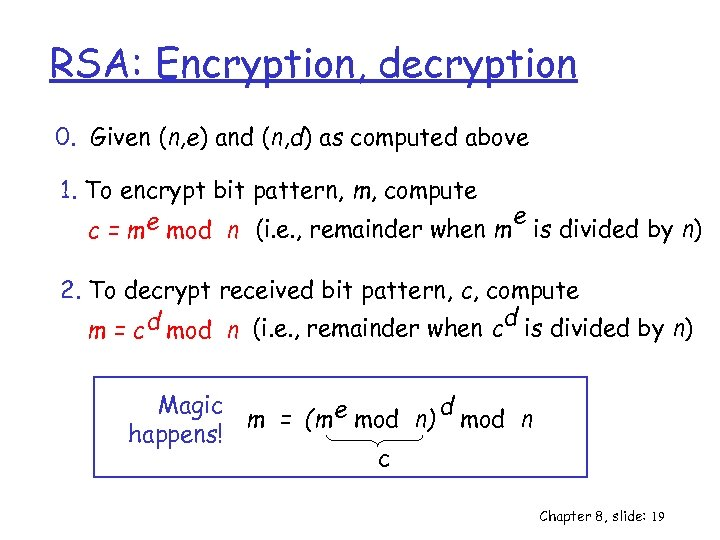RSA: Encryption, decryption 0. Given (n, e) and (n, d) as computed above 1. To encrypt bit pattern, m, compute e mod n (i. e. , remainder when m e is divided by n) c=m 2. To decrypt received bit pattern, c, compute d m = c d mod n (i. e. , remainder when c is divided by n) Magic d m = (m e mod n) mod n happens! c Chapter 8, slide: 19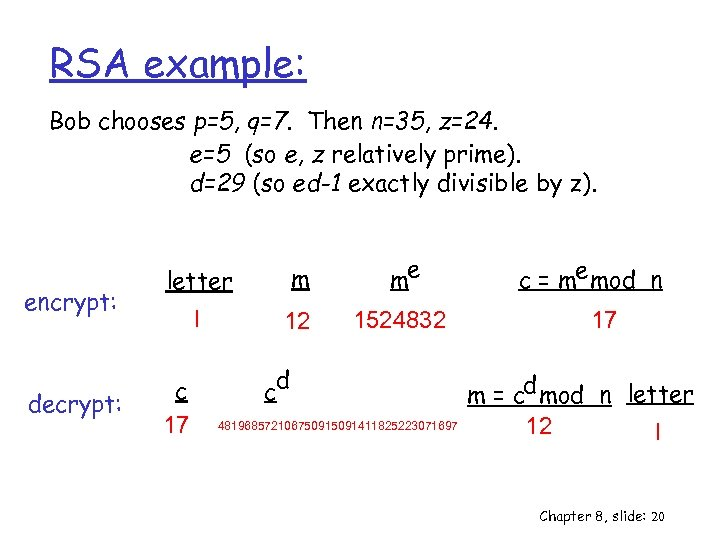RSA example: Bob chooses p=5, q=7. Then n=35, z=24. e=5 (so e, z relatively prime). d=29 (so ed-1 exactly divisible by z). encrypt: decrypt: letter m me l 12 1524832 c 17 d c 48196857210675091411825223071697 c = me mod n 17 m = cd mod n letter 12 l Chapter 8, slide: 20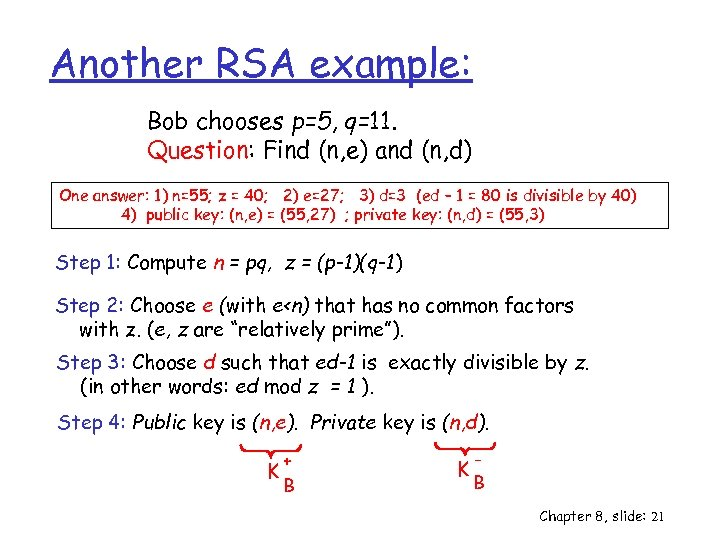Another RSA example: Bob chooses p=5, q=11. Question: Find (n, e) and (n, d) One answer: 1) n=55; z = 40; 2) e=27; 3) d=3 (ed – 1 = 80 is divisible by 40) 4) public key: (n, e) = (55, 27) ; private key: (n, d) = (55, 3) Step 1: Compute n = pq, z = (p-1)(q-1) Step 2: Choose e (with e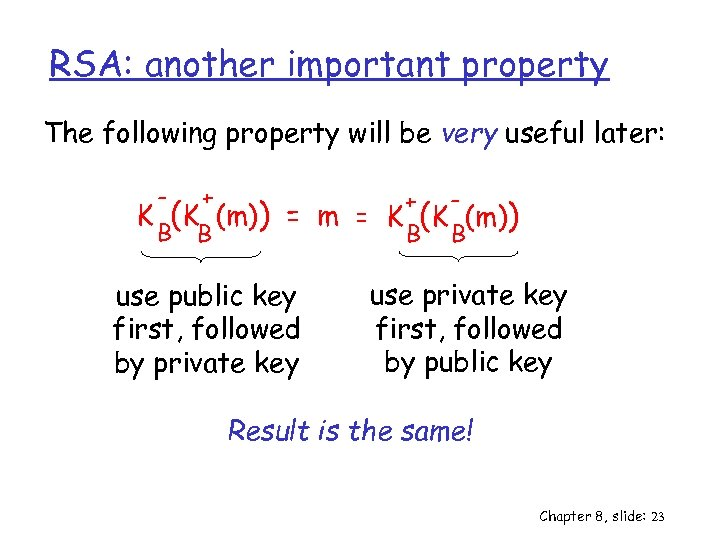RSA: another important property The following property will be very useful later: - + B B K (K (m)) + = m = K (K (m)) B B use public key first, followed by private key use private key first, followed by public key Result is the same! Chapter 8, slide: 23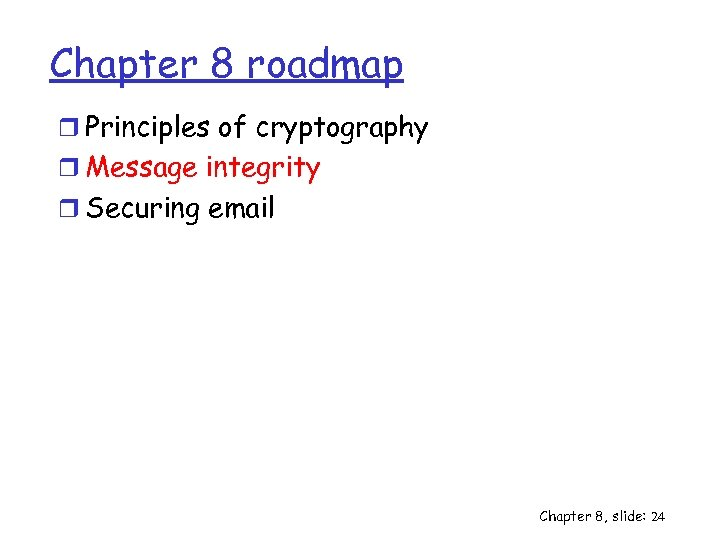Chapter 8 roadmap r Principles of cryptography r Message integrity r Securing email Chapter 8, slide: 24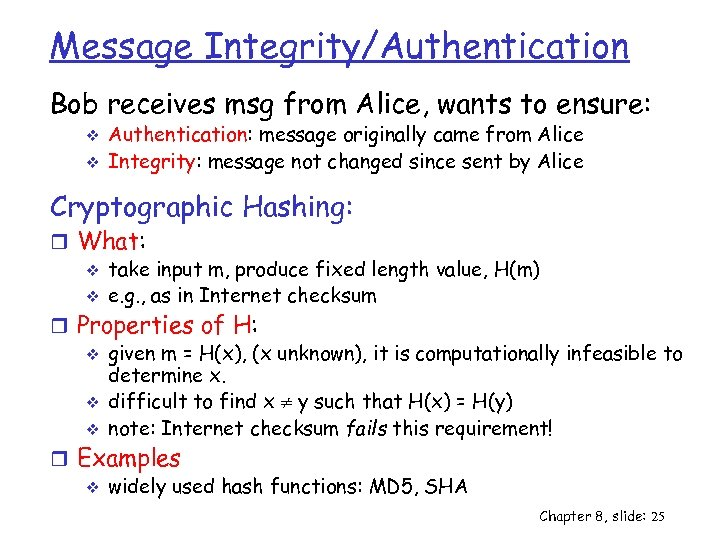Message Integrity/Authentication Bob receives msg from Alice, wants to ensure: v v Authentication: message originally came from Alice Integrity: message not changed since sent by Alice Cryptographic Hashing: r What: v take input m, produce fixed length value, H(m) v e. g. , as in Internet checksum r Properties of H: v given m = H(x), (x unknown), it is computationally infeasible to determine x. v difficult to find x y such that H(x) = H(y) v note: Internet checksum fails this requirement! r Examples v widely used hash functions: MD 5, SHA Chapter 8, slide: 25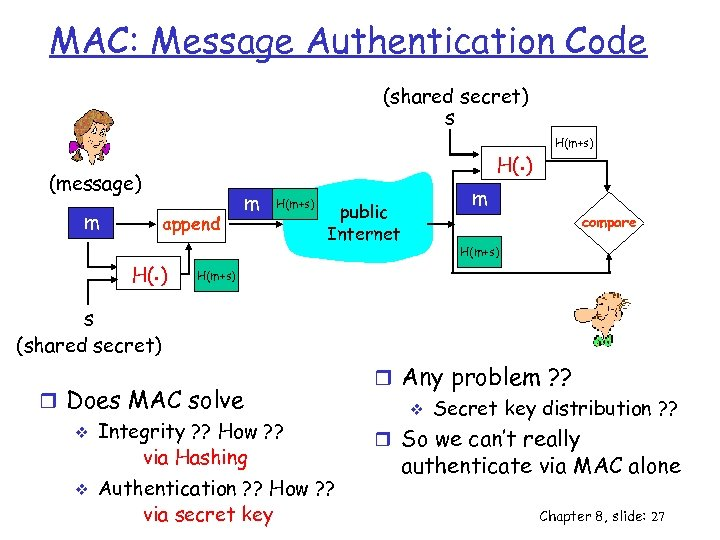MAC: Message Authentication Code (shared secret) s H(. ) (message) m append H(. ) m H(m+s) public Internet H(m+s) m compare H(m+s) s (shared secret) r Does MAC solve v Integrity ? ? How ? ? via Hashing v Authentication ? ? How ? ? via secret key r Any problem ? ? v Secret key distribution ? ? r So we can’t really authenticate via MAC alone Chapter 8, slide: 27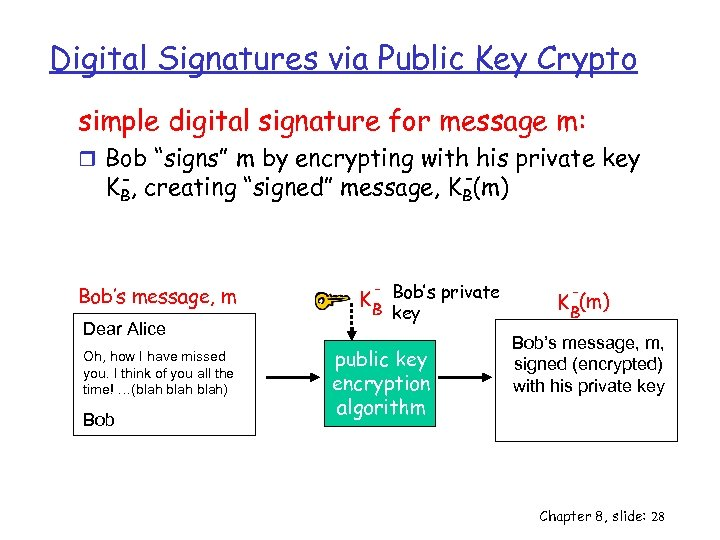Digital Signatures via Public Key Crypto simple digital signature for message m: r Bob “signs” m by encrypting with his private key K -, creating “signed” message, K -(m) B Bob’s message, m Dear Alice Oh, how I have missed you. I think of you all the time! …(blah) Bob B K B Bob’s private key public key encryption algorithm - K B(m) Bob’s message, m, signed (encrypted) with his private key Chapter 8, slide: 28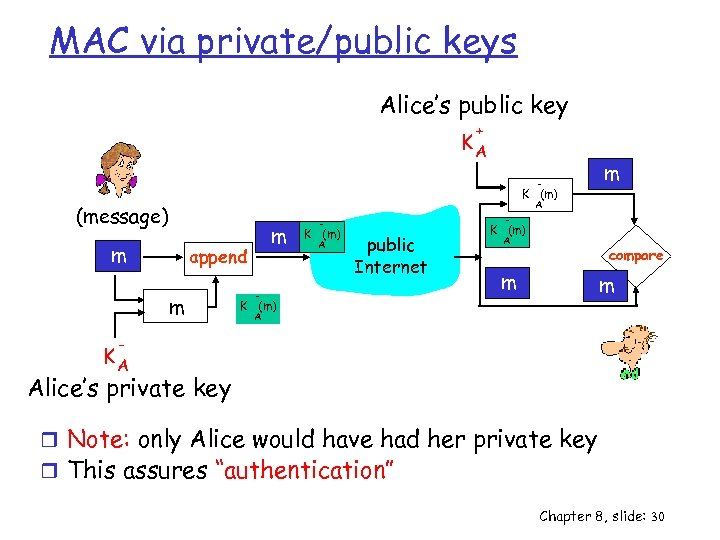MAC via private/public keys Alice’s public key + KA - K (m) m A (message) m m append m - - K (m) A public Internet - K (m) A compare m m K (m) A - KA Alice’s private key r Note: only Alice would have had her private key r This assures “authentication” Chapter 8, slide: 30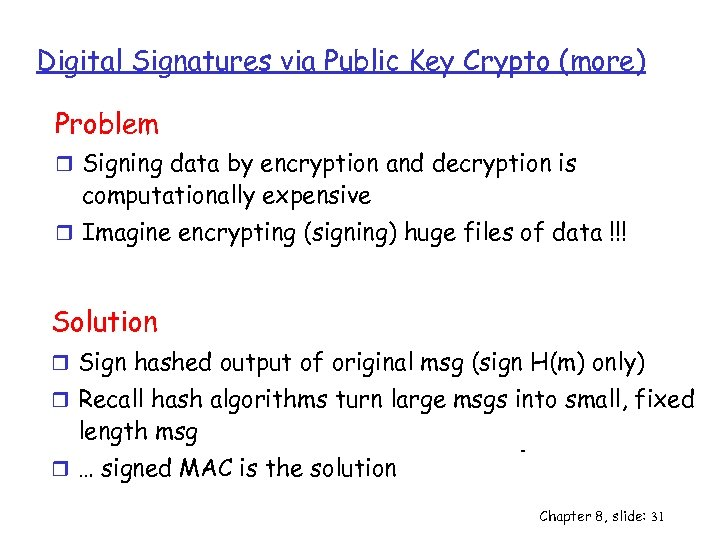Digital Signatures via Public Key Crypto (more) Problem r Signing data by encryption and decryption is computationally expensive r Imagine encrypting (signing) huge files of data !!! Solution r Sign hashed output of original msg (sign H(m) only) r Recall hash algorithms turn large msgs into small, fixed length msg r … signed MAC is the solution Chapter 8, slide: 31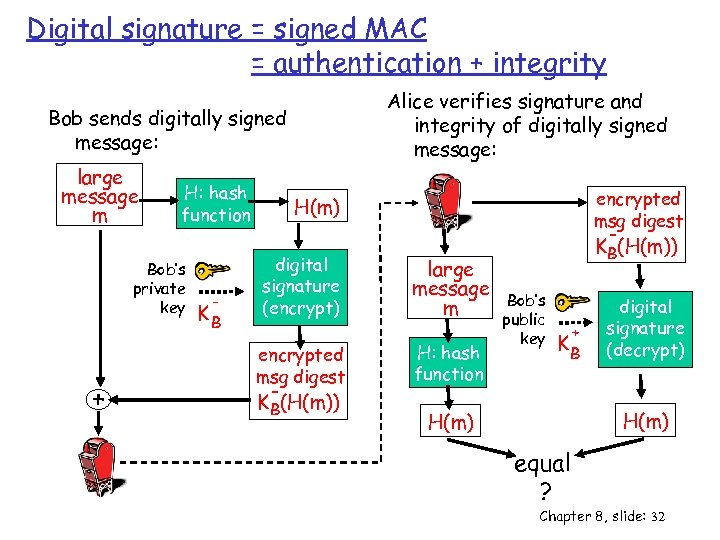Digital signature = signed MAC = authentication + integrity Alice verifies signature and integrity of digitally signed message: Bob sends digitally signed message: large message m H: hash function Bob’s private key + - KB encrypted msg digest H(m) digital signature (encrypt) encrypted msg digest KB(H(m)) large message m H: hash function KB(H(m)) Bob’s public key + KB digital signature (decrypt) H(m) equal ? Chapter 8, slide: 32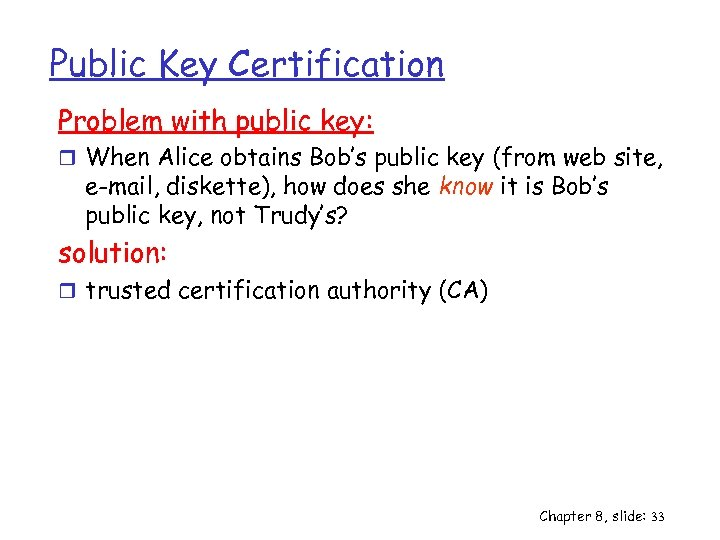Public Key Certification Problem with public key: r When Alice obtains Bob’s public key (from web site, e-mail, diskette), how does she know it is Bob’s public key, not Trudy’s? solution: r trusted certification authority (CA) Chapter 8, slide: 33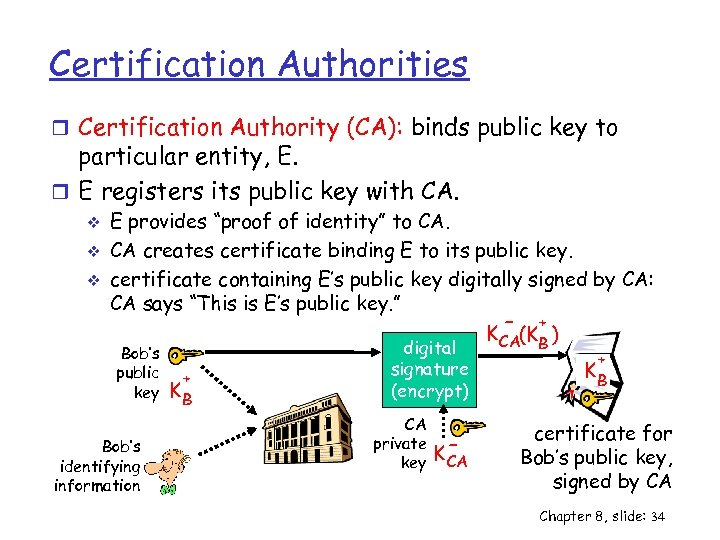Certification Authorities r Certification Authority (CA): binds public key to particular entity, E. r E registers its public key with CA. v v v E provides “proof of identity” to CA. CA creates certificate binding E to its public key. certificate containing E’s public key digitally signed by CA: CA says “This is E’s public key. ” - + K CA(KB ) Bob’s public key Bob’s identifying information + KB digital signature (encrypt) CA private key K- CA + KB certificate for Bob’s public key, signed by CA Chapter 8, slide: 34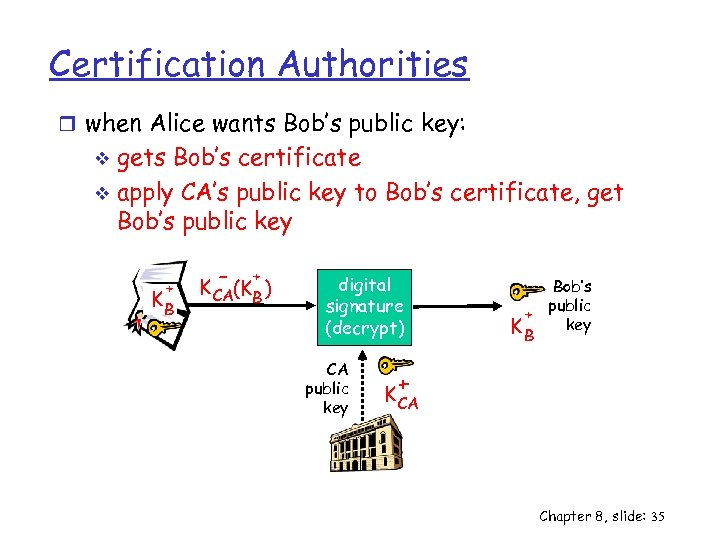Certification Authorities r when Alice wants Bob’s public key: gets Bob’s certificate v apply CA’s public key to Bob’s certificate, get Bob’s public key v + KB - + K CA(KB ) digital signature (decrypt) CA public key Bob’s public + key KB + K CA Chapter 8, slide: 35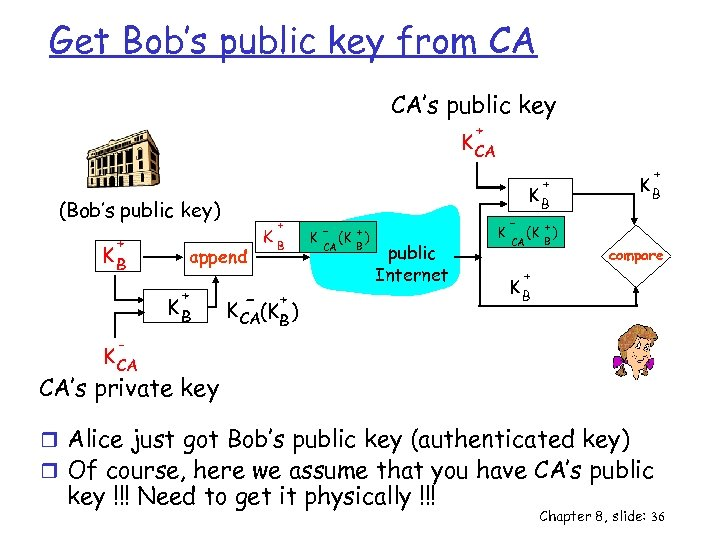Get Bob’s public key from CA CA’s public key + K CA (Bob’s public key) + KB append + KB - K + B K - (K + ) B CA public Internet + K CA(KB ) K + KB CA (K + ) B compare + KB - K CA CA’s private key r Alice just got Bob’s public key (authenticated key) r Of course, here we assume that you have CA’s public key !!! Need to get it physically !!! Chapter 8, slide: 36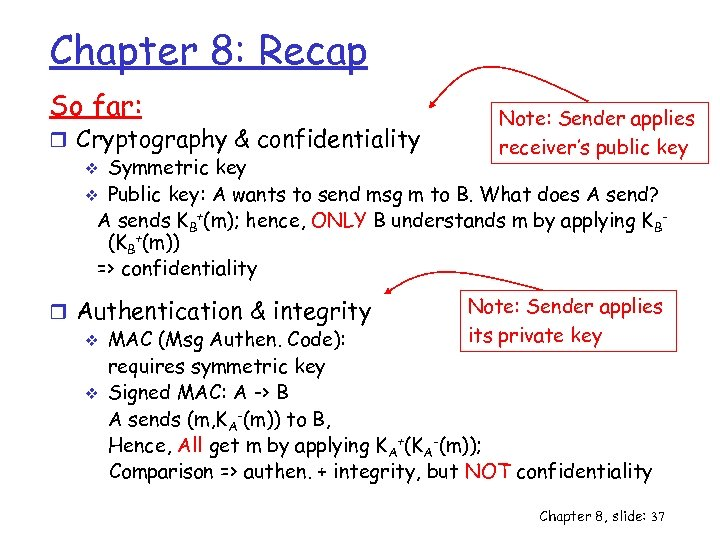Chapter 8: Recap So far: Note: Sender applies receiver’s public key r Cryptography & confidentiality v Symmetric key v Public key: A wants to send msg m to B. What does A send? A sends KB+(m); hence, ONLY B understands m by applying KB(KB+(m)) => confidentiality Note: Sender applies r Authentication & integrity its private key v MAC (Msg Authen. Code): requires symmetric key v Signed MAC: A -> B A sends (m, KA-(m)) to B, Hence, All get m by applying KA+(KA-(m)); Comparison => authen. + integrity, but NOT confidentiality Chapter 8, slide: 37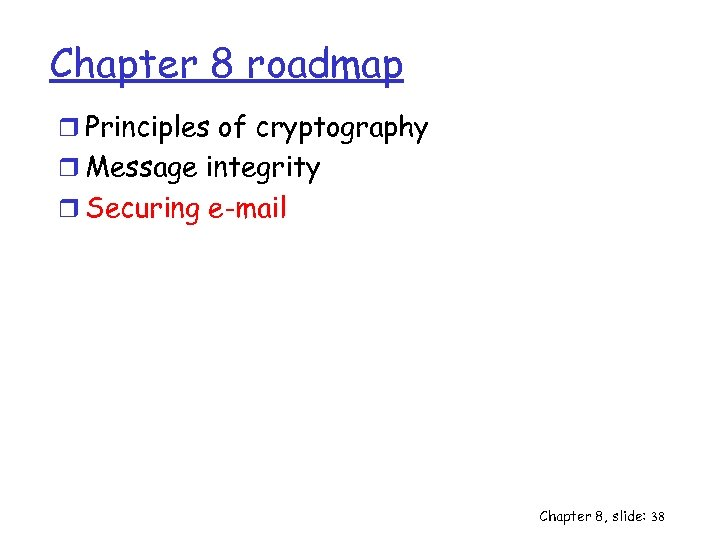Chapter 8 roadmap r Principles of cryptography r Message integrity r Securing e-mail Chapter 8, slide: 38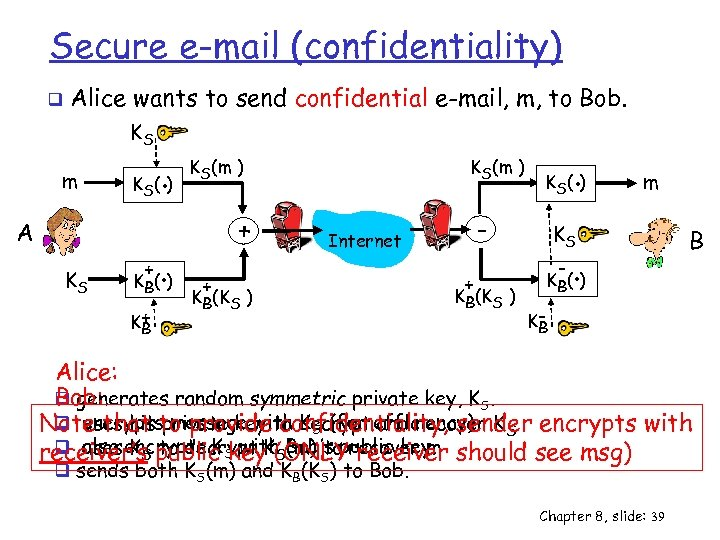Secure e-mail (confidentiality) q Alice wants to send confidential e-mail, m, to Bob. KS m K (. ) S A KS + . K B( ) K+ B KS(m ) + - Internet + KB(KS ) . K S( ) + KB(KS ) m KS - . B K B( ) KB Alice: Bob: q generates random symmetric private key, KS. q encryptsprivate key confidentiality, sender uses his message with KS (for and recover Note that to provideto decrypt efficiency) KS encrypts with q also encrypts K key. K(ONLYrecover m uses KS to decrypt (m) to receiver’s public S with. SBob’s public key. should see msg) q sends both KS(m) and KB(KS) to Bob. Chapter 8, slide: 39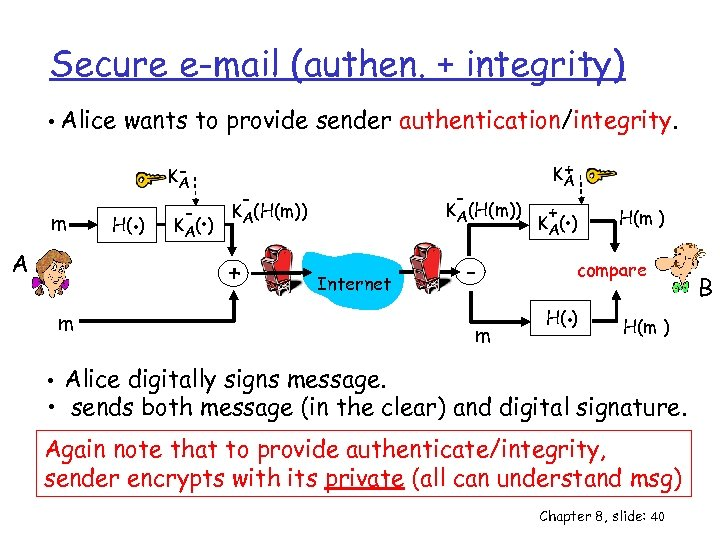Secure e-mail (authen. + integrity) • Alice wants to provide sender authentication/integrity. m A . H( ) KA - . K A( ) - - KA(H(m)) + + KA Internet m m + . K A( ) H(m ) compare . H( ) H(m ) • Alice digitally signs message. • sends both message (in the clear) and digital signature. Again note that to provide authenticate/integrity, sender encrypts with its private (all can understand msg) Chapter 8, slide: 40 B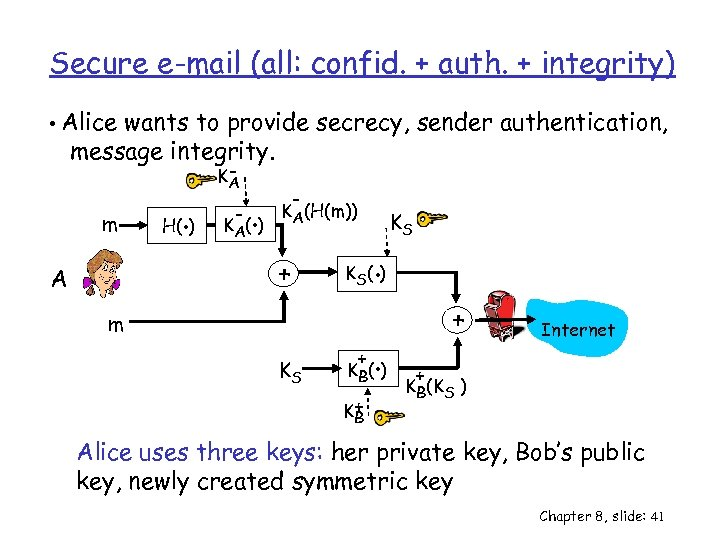Secure e-mail (all: confid. + auth. + integrity) • Alice wants to provide secrecy, sender authentication, message integrity. m . H( ) KA - . K A( ) - KA(H(m)) + A . K S( ) m KS KS + . K B( ) K+ B + Internet + KB(KS ) Alice uses three keys: her private key, Bob’s public key, newly created symmetric key Chapter 8, slide: 41The end of new material! Final Review on Thursday! Chapter 8, slide: 42